• 2020-10-09 14:32:21

## 引言

XGBoost用于建模，SHAP用于模型的可视化解释。
多分类实例：
https://blog.csdn.net/weixin_45520028/article/details/109003707
XGBoost原理参考推荐：
https://zhuanlan.zhihu.com/p/75217528
https://www.cnblogs.com/wj-1314/p/9402324.html
官方参数解释
XGB中文解释
SHAP参考推荐：
https://blog.csdn.net/weixin_45520028/article/details/108857908
另外，多分类模型解释参考：
https://developer.aliyun.com/article/715920

# 1 数据准备

XGB准备原始数据为一个dataframe，其中一列为输出的结果值，其他列为模型的特征值。

• 输出结果值：
二分类模型：只能为’0’或’1’
多分类模型：从’0’开始的数字
• 模型特征值：
必须为数值型，如整数、小数；如果为字符，如中文描述，需要先进行转换。

字符转数值方法：
法一：直接转稀疏矩阵：

# 将col1和col2两列的内容转稀疏矩阵。一列中有n个不同的值，该列将转成n列稀疏矩阵
pd.get_dummies(data, drop_first=True, columns=['col1','col2'])


法二：函数转数值LabelEncoder

# data为原数据dataframe，data_deal为需要转换的dataframe
data_deal = data[['col1', 'col2',
'col3', ......]]
# 先转为str格式，中/英文都需要先转str便于后续函数处理
data_deal['col1'] = data_deal['col1'].astype(str)
# ......

# 字符转换为数值
# features用于记录转换完成的数值
# feature_tables用于记录完整的原字符和转换后数值
features = []
feature_tables = []

for i in range(0, data_deal.shape):
label_encoder = LabelEncoder()
feature = label_encoder.fit_transform(data_deal.iloc[:, i])
feature_table = label_encoder.inverse_transform(feature)
features.append(feature)
feature_tables.append(feature)
feature_tables.append(feature_table)
# 如需反转使用label_encoder.inverse_transform(feature)
data_deal2 = np.array(features)
data_deal2 = data_deal2.reshape(data_deal.shape, data_deal.shape)
data_deal3 = pd.DataFrame(data_deal2)

# data返回替换，完成数值型转换
data['col1'] = data_deal3.iloc[:, 0]
data['col2'] = data_deal3.iloc[:, 1]
data['col3'] = data_deal3.iloc[:, 2]
# ......

# 额外查看操作，不影响建模过程
# 查看字符-数字转换替换表
# 特征对应完整表
feature_tables = pd.DataFrame(np.array(feature_tables)).T
# 去重查看转换对照表
# subsidy_sensitive对应表
fea_sub_sens = feature_tables.iloc[:,:2].drop_duplicates().reset_index(drop=True).sort_values(by=1)
# order_subsidy_sensitive_level对应表
fea_ord_sub_sens = feature_tables.iloc[:,2:4].drop_duplicates().reset_index(drop=True).sort_values(by=3)
# 二second_level_stage_net对应表
fea_sec_lv_stage = feature_tables.iloc[:,4:6].drop_duplicates().reset_index(drop=True).sort_values(by=5)
# ......


法三：函数转数值OneHotEncoder
输出与法一类似。

原数据抽样
根据需要使用，可以选择随机抽样，抽出不同分类的数据总行数相同的分类组。

# 结果1、结果2、结果0抽取同数量组数
data_s1 = data[data['col_result'] == 1].sample(n=650000, random_state=1)
data_s2 = data[data['col_result'] == 2].sample(n=650000, random_state=1)
data_s0 = data[data['col_result'] == 0].sample(n=650000, random_state=1)

# 拼接新的数据集
data = pd.concat([data_s1, data_s2, data_s0], ignore_index=True, axis=0)


# 2 数据处理

准备XGB的输入和输出数据，训练集和测试集。

# XGBoost建模数据准备，输入特征值和输出结果
data_result = data.iloc[:, 17]
data_input = data.iloc[:, 1:17]

# 准备xgb训练输入、测试输入、训练输出、测试输出
# 测试集选1%的原数据规模
train_x, test_x, train_y, test_y = train_test_split(data_input, data_result, test_size=0.01, random_state=0)


# 3 XGB模型

# xgb处理训练集和测试集
dtrain = xgb.DMatrix(train_x, label=train_y)
dtest = xgb.DMatrix(test_x)

# 参数
params = {'booster': 'gbtree',
'objective': 'multi:softprob', #多分类'multi:softmax'返回预测的类别(不是概率)，'multi:softprob'返回概率
'num_class': 3,
'eval_metric': 'merror', #二分类用’auc‘，多分类用'mlogloss'或'merror'
'max_depth': 7,
'lambda': 15,
'subsample': 0.75,
'colsample_bytree': 0.75,
'min_child_weight': 1,
'eta': 0.025,
'seed': 0,
'silent': 1,
'gamma': 0.15,
'learning_rate': 0.01}

watchlist = [(dtrain, 'train')]

# 建模与预测:NUM_BOOST_round迭代次数和数的个数一致
model = xgb.train(params, dtrain, num_boost_round=50, evals=watchlist)
ypred = model.predict(dtest)


4 模型评估

# XGBoost使用笔记

## 1 XGBoost模型参数解释

'objective’参数解释

“reg:linear”  # 线性回归模型
“reg:logistic”  # 逻辑回归模型
“binary:logistic”  # 二分类的逻辑回归模型，输出为概率。
“binary:logitraw”  # 二分类的逻辑回归问题，输出的结果为wTx
“count:poisson”  # 计数问题的poisson回归，输出结果为poisson分布。在poisson回归中，max_delta_step的缺省值为0.7
“multi:softmax”  # 多分类模型，同时需设置参数num_class（分类个数），输出为分类结果
“multi:softprob”  # 和softmax一样，但输出的是概率，为n * m 的向量(n行，m分类数)
“rank:pairwise”  # set XGBoost to do ranking task by minimizing the pairwise loss


## 2 XGBoost通用参数解释机器学习 python
更多相关内容
• softmax 回归(softmax regression)其实是 logistic 回归的一般形式，logistic 回归用于二分类，而 softmax 回归用于多分类，关于 logistic 回归可以看我的这篇博客????机器学习笔记九——线性模型原理以及python实现...

# 2、Softmax回归模型

\quad \quad Softmax回归跟线性回归一样将输入特征与权重做线性叠加。与线性回归的一个主要不同在于，Softmax回归的输出值个数等于标签里的类别数。比如一共有4种特征和3种输出动物类别(狗、猫、鸡），则权重包含12个标量（带下标的 w w ）、偏差包含3个标量（带下标的 b b ），且对每个输入计算 o 1 , o 2 , o 3 o_1, o_2, o_3 这3个输出：

o 1 = x 1 w 11 + x 2 w 21 + x 3 w 31 + x 4 w 41 + b 1 , o 2 = x 1 w 12 + x 2 w 22 + x 3 w 32 + x 4 w 42 + b 2 , o 3 = x 1 w 13 + x 2 w 23 + x 3 w 33 + x 4 w 43 + b 3 . \begin{aligned} o_1 &= x_1 w_{11} + x_2 w_{21} + x_3 w_{31} + x_4 w_{41} + b_1,\\ o_2 &= x_1 w_{12} + x_2 w_{22} + x_3 w_{32} + x_4 w_{42} + b_2,\\ o_3 &= x_1 w_{13} + x_2 w_{23} + x_3 w_{33} + x_4 w_{43} + b_3. \end{aligned}
最后，再对这些输出值进行Softmax函数运算\quad \quad 图3.2用神经网络图描绘了上面的计算。softmax回归同线性回归一样，也是一个单层神经网络。由于每个输出 o 1 , o 2 , o 3 o_1, o_2, o_3 的计算都要依赖于所有的输入 x 1 , x 2 , x 3 , x 4 x_1, x_2, x_3, x_4 ，所以softmax回归的输出层也是一个全连接层。

# 3、Softmax函数

\quad \quad Softmax用于多分类过程中，它将多个神经元的输出(比如 o 1 , o 2 , o 3 o_1, o_2, o_3 )映射到（0,1）区间内，可以看成概率来理解，从而来进行多分类！它通过下式将输出值变换成值为正且和为1的概率分布：

y ^ 1 , y ^ 2 , y ^ 3 = softmax ( o 1 , o 2 , o 3 ) , \hat{y}_1, \hat{y}_2, \hat{y}_3 = \text{softmax}(o_1, o_2, o_3),

其中

y ^ 1 = exp ⁡ ( o 1 ) ∑ i = 1 3 exp ⁡ ( o i ) , y ^ 2 = exp ⁡ ( o 2 ) ∑ i = 1 3 exp ⁡ ( o i ) , y ^ 3 = exp ⁡ ( o 3 ) ∑ i = 1 3 exp ⁡ ( o i ) . \hat{y}_1 = \frac{ \exp(o_1)}{\sum_{i=1}^3 \exp(o_i)},\quad \hat{y}_2 = \frac{ \exp(o_2)}{\sum_{i=1}^3 \exp(o_i)},\quad \hat{y}_3 = \frac{ \exp(o_3)}{\sum_{i=1}^3 \exp(o_i)}.

容易看出 y ^ 1 + y ^ 2 + y ^ 3 = 1 \hat{y}_1 + \hat{y}_2 + \hat{y}_3 = 1 0 ≤ y ^ 1 , y ^ 2 , y ^ 3 ≤ 1 0 \leq \hat{y}_1, \hat{y}_2, \hat{y}_3 \leq 1 ，因此 y ^ 1 , y ^ 2 , y ^ 3 \hat{y}_1, \hat{y}_2, \hat{y}_3 是一个合法的概率分布。这时候，如果 y ^ 2 = 0.8 \hat{y}_2=0.8 ，不管 y ^ 1 \hat{y}_1 y ^ 3 \hat{y}_3 的值是多少，我们都知道图像类别为猫的概率是80%。此外，我们注意到

argmax ⁡ o i = argmax ⁡ y ^ i , \operatorname{argmax} o_i = \operatorname{argmax} \hat y_i,

因此softmax运算不改变预测类别输出。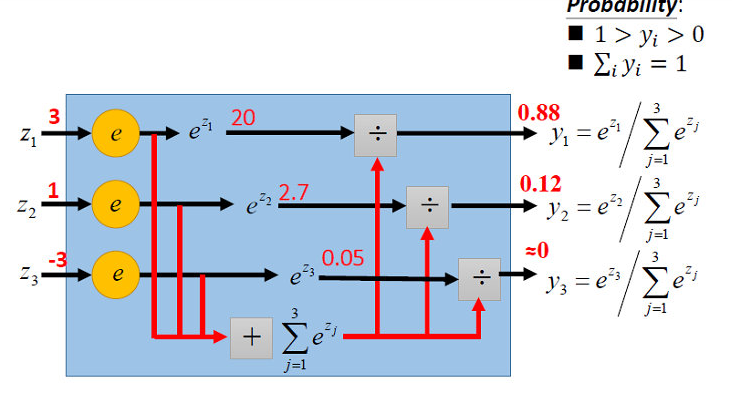# 4、Softmax回归的决策函数

W = [ w 11 w 12 w 13 w 14 w 21 w 22 w 23 w 24 w 31 w 32 w 33 w 34 ] , b = [ b 1 b 2 b 3 ] , \boldsymbol{W} = \begin{bmatrix} w_{11} & w_{12} & w_{13} & w_{14} \\ w_{21} & w_{22} & w_{23}& w_{24} \\ w_{31} & w_{32} & w_{33} & w_{34} \end{bmatrix},\quad \boldsymbol{b} = \begin{bmatrix} b_1 & b_2 & b_3 \end{bmatrix},

设高和宽分别为2个像素的图像样本 i i 的特征为

x ( i ) = [ x 1 ( i ) x 2 ( i ) x 3 ( i ) x 4 ( i ) ] , \boldsymbol{x}^{(i)} = \begin{bmatrix}x_1^{(i)} & x_2^{(i)} & x_3^{(i)} & x_4^{(i)}\end{bmatrix},

输出层的输出为

o ( i ) = [ o 1 ( i ) o 2 ( i ) o 3 ( i ) ] , \boldsymbol{o}^{(i)} = \begin{bmatrix}o_1^{(i)} & o_2^{(i)} & o_3^{(i)}\end{bmatrix},

预测为狗、猫或鸡的概率分布为

y ^ ( i ) = [ y ^ 1 ( i ) y ^ 2 ( i ) y ^ 3 ( i ) ] . \boldsymbol{\hat{y}}^{(i)} = \begin{bmatrix}\hat{y}_1^{(i)} & \hat{y}_2^{(i)} & \hat{y}_3^{(i)}\end{bmatrix}.

Softmax回归对样本 i i 分类的矢量计算表达式为

o ( i ) = x ( i ) W T + b , y ^ ( i ) = softmax ( o ( i ) ) = exp ⁡ ( o i ) ∑ i = 1 3 exp ⁡ ( o i ) . \begin{aligned} \boldsymbol{o}^{(i)} &= \boldsymbol{x}^{(i)} \boldsymbol{W}^T + \boldsymbol{b},\\ \boldsymbol{\hat{y}}^{(i)} &= \text{softmax}(\boldsymbol{o}^{(i)})= \frac{ \exp(o_i)}{\sum_{i=1}^3 \exp(o_i)}. \end{aligned}
则Softmax回归的决策函数可以表示为：

y ^ = a r g m a x y ^ ( i ) \hat{\boldsymbol{y}}=\boldsymbol{argmax}\quad \boldsymbol {\hat{y}}^{(i)}

# 5、参数估计

## 5.1 损失函数

\quad \quad 给定N个训练样本 { ( x ( n ) , y ( n ) ) } n = 1 N \{(x^{(n)},y^{(n)})\}_{n=1}^N ,样本一共有C个类别，用C维的one-hot向量 y ∈ { 0 , 1 } C y\in\{0,1\}^C 来表示类别标签。【对于类别c,其向量表示为 y c = [ I ( 1 = c ) , I ( 2 = c ) , . . . , I ( C = c ) ] T y_c=[I(1=c),I(2=c),...,I(C=c)]^T ,其中 I ( . ) I(.) 是指示函数】

L ( W , b ) = − 1 N ∑ n = 1 N ∑ c = 1 C y c ( n ) l o g y ^ c ( n ) = − 1 N ∑ n = 1 N ( y ( n ) ) T l o g y ^ ( n ) L(\boldsymbol{W} ,\boldsymbol{b})=-\frac1N\sum_{n=1}^N\sum_{c=1}^C y_c^{(n)}log\hat{y}_c^{(n)}=-\frac1N\sum_{n=1}^N (y^{(n)})^Tlog\hat{y}^{(n)}
其中， y ^ ( n ) = S o f t m a x ( x ( n ) W T + b ) \hat{y}^{(n)}=Softmax(\boldsymbol{x}^{(n)} \boldsymbol{W}^T + \boldsymbol{b}) 为样本 x ( n ) x^{(n)} 在每个类别的后验概率。

当样本只有一个标签时，我们可以对损失函数简化，简化如下：

\quad \quad 当每个样本的真实标签 y ( n ) y^{(n)} 只有一个标签，即是一个C维one-hot向量：若样本属于i类，则只有第i维是1，其余都是0（ c ∈ [ 1 , C ] ; n ∈ [ 1 , N ] ) c\in[1,C];n\in[1,N]) 。所以
L ( W , b ) = − 1 N ∑ n = 1 N ( y ( n ) ) T l o g y ^ ( n ) = − 1 N ∑ n = 1 N l o g y ^ y ( n ) ( n ) L(\boldsymbol{W} ,\boldsymbol{b})=-\frac1N\sum_{n=1}^N (y^{(n)})^Tlog\hat{y}^{(n)}=-\frac1N\sum_{n=1}^N log\hat{y}_{y^{(n)}}^{(n)}

其中，假设第n个样本的真实标签为第i类即 y ( n ) y^{(n)} 的第i维为1，则 y ^ y ( n ) ( n ) \hat{y}_{y^{(n)}}^{(n)} 为样本预测值中第i维的值。

\quad \quad 从另一个角度来看，我们知道最小化损失函数 L ( W , b ) L(W,b) 等价于最大化 exp ⁡ ( − n L ( W , b ) ) = ∏ i = 1 n y ^ y ( i ) ( i ) \exp(-nL(W,b))=\prod_{i=1}^n \hat y_{y^{(i)}}^{(i)} ，即最小化交叉熵损失函数等价于最大化训练数据集所有标签类别的联合预测概率。

## 5.2 梯度估计

### 5.2.1 Softmax函数的导数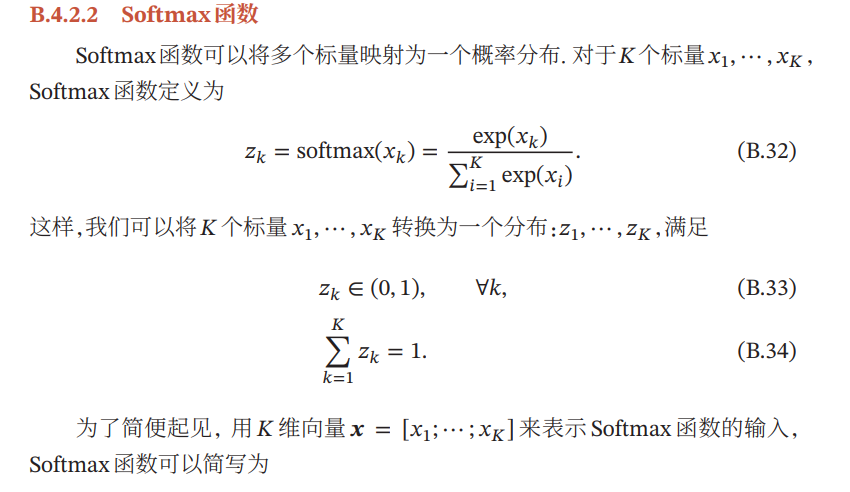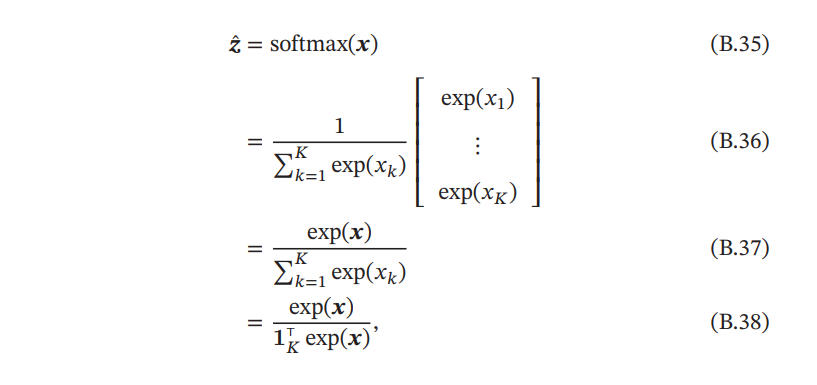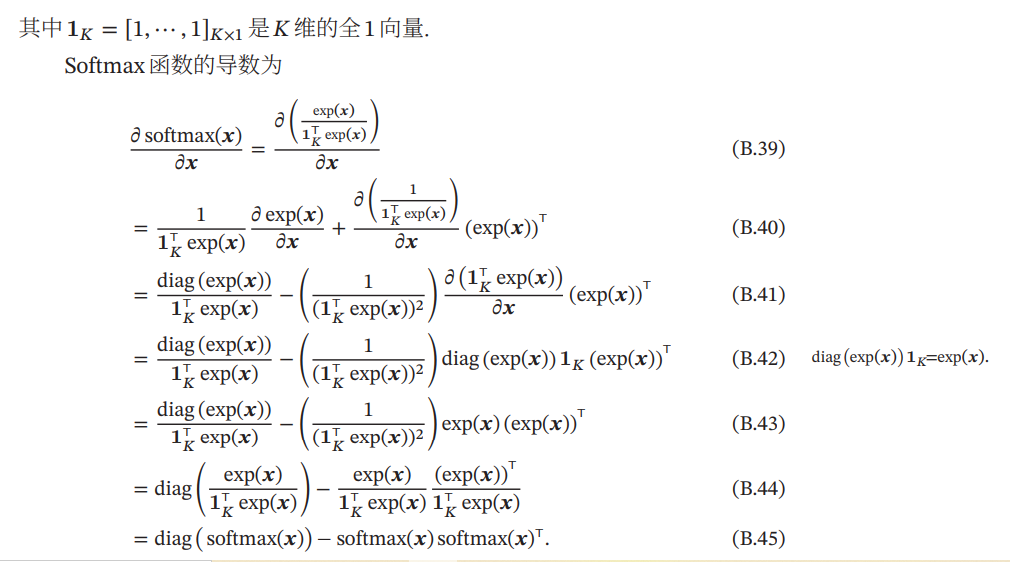来源于神经网络与深度学习的附录

### 5.2.2 梯度

损失函数 L ( W ) L(W) 关于W的梯度为：
∂ L ( W ) ∂ W = − 1 N ∑ n = 1 N x ( n ) ( y ( n ) − y ^ ( n ) ) T \frac{ \partial L(W) }{\partial W}=-\frac 1N\sum_{n=1}^Nx(n)(y^{(n)}-\hat{y}^{(n)})^T

采用梯度下降法，Softmax回归的训练过程为：

1）初始化 W 0 = 0 W_0=0 【在神经网络中，不能全部初始化为0，可随机初始化】
2) 迭代更新：
W t + 1 = W t + α ( 1 N ∑ n = 1 N x ( n ) ( y ( n ) − y ^ ( n ) ) T ) W_{t+1}=W_t+\alpha(\frac 1N\sum_{n=1}^Nx(n)(y^{(n)}-\hat{y}^{(n)})^T)

预测

评价

# 7、Softmax回归的从零开始实现

我们将使用Fashion-MNIST数据集【简介】，进行图像多分类问题。

导入所需的包

import torch
import torchvision
import numpy as np
import sys
sys.path.append('E:\d2lzh_pytorch')#加上d2lzh_pytorch的路径
import d2lzh_pytorch as d2l



1、读取数据集

batch_size = 256#小批量进行读取


2、初始化模型参数

跟线性回归中的例子一样，我们将使用向量表示每个样本。已知每个样本输入是高和宽均为28像素的图像。模型的输入向量的长度是 28 × 28 = 784 28 \times 28 = 784 ：该向量的每个元素对应图像中每个像素。由于图像有10个类别，单层神经网络输出层的输出个数为10，因此softmax回归的权重和偏差参数分别为 784 × 10 784 \times 10 1 × 10 1 \times 10 的矩阵。

num_inputs = 784
num_outputs = 10
#随机初始化参数
W = torch.tensor(np.random.normal(0, 0.01, (num_inputs, num_outputs)), dtype=torch.float)
b = torch.zeros(num_outputs, dtype=torch.float)


为模型参数附上梯度。

W.requires_grad_(requires_grad=True)


3、定义Softmax函数

def softmax(X):
X_exp = X.exp()
partition = X_exp.sum(dim=1, keepdim=True)#行元素求和
return X_exp / partition  # 这里应用了广播机制


4、定义Softmax回归模型

def net(X):
return softmax(torch.mm(X.view((-1, num_inputs)), W) + b)


5、定义损失函数

def cross_entropy(y_hat, y):
return - torch.log(y_hat.gather(1, y.view(-1, 1)))


6、计算分类准确率

给定一个类别的预测概率分布y_hat，我们把预测概率最大的类别作为输出类别。如果它与真实类别y一致，说明这次预测是正确的。分类准确率即正确预测数量与总预测数量之比。

为了演示准确率的计算，下面定义准确率accuracy函数。其中y_hat.argmax(axis=1)返回矩阵y_hat每行中最大元素的索引，且返回结果与变量y形状相同。我们在“数据操作”一节介绍过，相等条件判别式(y_hat.argmax(axis=1) == y)是一个类型为ByteTensorTensor,我们用float()将其转换为值为0（相等为假）或1（相等为真）的浮点型Tensor

def accuracy(y_hat, y):
return (y_hat.argmax(dim=1) == y).float().mean().item()


7、评价模型net在数据集data_iter上的准确率。

# 本函数已保存在d2lzh_pytorch包中方便以后使用。该函数将被逐步改进：它的完整实现将在“图像增广”一节中描述
def evaluate_accuracy(data_iter, net):
acc_sum, n = 0.0, 0
for X, y in data_iter:
acc_sum += (net(X).argmax(dim=1) == y).float().sum().item()
n += y.shape
return acc_sum / n


8、训练模型

num_epochs, lr = 5, 0.1

# 本函数已保存在d2lzh_pytorch包中方便以后使用
def train_ch3(net, train_iter, test_iter, loss, num_epochs, batch_size,
params=None, lr=None, optimizer=None):
for epoch in range(num_epochs):
train_l_sum, train_acc_sum, n = 0.0, 0.0, 0
for X, y in train_iter:
y_hat = net(X)
l = loss(y_hat, y).sum()

# 梯度清零
if optimizer is not None:
elif params is not None and params.grad is not None:
for param in params:

l.backward()
if optimizer is None:
d2l.sgd(params, lr, batch_size)
else:
optimizer.step()  # “softmax回归的简洁实现”一节将用到

train_l_sum += l.item()
train_acc_sum += (y_hat.argmax(dim=1) == y).sum().item()
n += y.shape
test_acc = evaluate_accuracy(test_iter, net)
print('epoch %d, loss %.4f, train acc %.3f, test acc %.3f'
% (epoch + 1, train_l_sum / n, train_acc_sum / n, test_acc))

train_ch3(net, train_iter, test_iter, cross_entropy, num_epochs, batch_size, [W, b], lr)


epoch 1, loss 0.7852, train acc 0.750, test acc 0.789
epoch 2, loss 0.5707, train acc 0.813, test acc 0.813
epoch 3, loss 0.5258, train acc 0.826, test acc 0.818
epoch 4, loss 0.5008, train acc 0.832, test acc 0.824
epoch 5, loss 0.4843, train acc 0.838, test acc 0.827

9、预测

for X, y in test_iter:
break

true_labels = d2l.get_fashion_mnist_labels(y.asnumpy())
pred_labels = d2l.get_fashion_mnist_labels(net(X).argmax(axis=1).asnumpy())
titles = [true + '\n' + pred for true, pred in zip(true_labels, pred_labels)]

d2l.show_fashion_mnist(X[0:9], titles[0:9])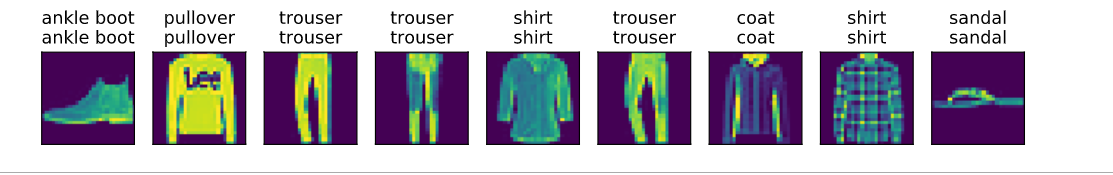参考资料：
1、https://blog.csdn.net/weixin_38169413/article/details/103598534
2、神经网络与深度学习
3、动手学深度学习

展开全文深度学习 Softmax回归
• 5、分类报告 from sklearn.metrics import classification_report y_true = [0, 1, 2, 2, 0] y_pred = [0, 0, 2, 1, 0] target_names = [‘class 0’, ‘class 1’, ‘class 2’] print(classification_report...

## 1、准确率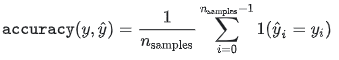metrics.accuracy_score(y_true=y_true, y_pred=y_pred)

## 2、平均准确率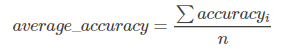针对不平衡数据，对n个类，分别计算每个类别的准确率，然后求平均值。
metrics.average_precision_score(y_true=y_true, y_score=y_pred)

## 3、基于相似度的评价指标

### 3.1 log-loss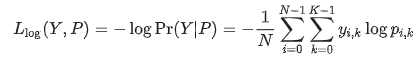指示矩阵Y(N*K)，yi,k=1如果第i个数据属于第k类，否则yi,k=0。pi,k为预测概率。
metrics.log_loss(y_true,y_pred)

### 3.2 hamming_loss

metrics.hamming_loss(y_true, y_pred)

### 3.3 jaccard_similarity_score

metrics.jaccard_similarity_score(y_true, y_pred)

## 4、混淆矩阵

在混淆矩阵中的i，j指的是观察的数目i，预测为j。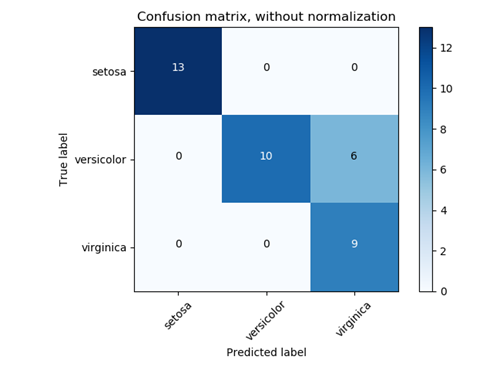metrics.confusion_matrix(y_true, y_pred)

## 5、分类报告

from sklearn.metrics import classification_report
y_true = [0, 1, 2, 2, 0]
y_pred = [0, 0, 2, 1, 0]
target_names = [‘class 0’, ‘class 1’, ‘class 2’]
print(classification_report(y_true, y_pred, target_names=target_names))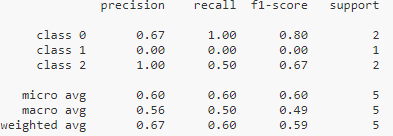展开全文• ## 应用XGboost实现多分类模型实践

万次阅读 热门讨论 2019-04-04 16:44:45
本文把XGBoost集成算法模型，应用在工业生产中，分析生产过程数据，提高...实验学习目标采用XGBoost多分类multi:softprob输出概率，在8000个样本条件下，训练模型，达到62%准确率。本文分享了实验中关键点和填过的坑。

在应用XGBoost算法进行多分类应用时，发生了如下错误：
XGBoostError: b’value 0 for Parameter num_class should be greater equal to 1’
意思是，参数num_class的xgboost sklearn包装值0应该大于1。
忽然，我理解了参数及其设置，这里的”num_class“参数，与”objective“参数匹配，本案例是多分类应用，那么”objective“参数设置为”multi:softmax“，同时，”num_class“参数设置为”4“（本文用到的4个分类，0、1、2、3，分别代表着0-正常,1- 抽油杆断,2- 供液不足,3- 气影响，参考）。

# 1. 输入数据

本案例数据源为csv格式（csv格式为每个数据由逗号“，”分隔）。

0.29,0.31,32.8,92.5,22,20,0.7750820447097237,0.5326322957198866
0.61,0.76,7.8,84.4,42,40,0.6650599517190358,0.47773242152197465
0.21,0.23,19.8,84.8,31,30,0.6798212287221709,0.6725660513935667
0.25,0.28,88.1,94.3,75,74,0.529969222169283,0.5878974216397194
0.45,0.48,16.5,99.9,77,75,0.6958968659268767,0.7047297521312826
0.4,0,39.2,94.2,36,32,0.7301196680207003,0.48968041620245173


数据的含义分别是“油压”、“套压”、“产液量”、“含水率”、“上行电流”、“下行电流”，以及数据特征变换值“欧几里得距离”、“功图面积与基准面积的比值”。

注：输入数据是数值型。XGBoost 和 CatBoost 以及 LGBM 算法不同，XGBoost 本身无法处理分类变量，而是像随机森林一样，只接受数值数据。因此在将分类数据传入 XGBoost 之前，必须通过各种编码方式：例如标记编码、均值编码或独热编码对数据进行处理。
由于每个井的井号是非数值型，而且只是个分类标识号，无数值意义。为了区分各个井的差别，体现相同的井，使用井的描述参数，例如：泵径、沉没度、下泵深度等等。

# 2. 模型及其参数

XGBoost模型objective说明：

• “reg:linear” —— 线性回归。
• “reg:logistic”—— 逻辑回归。
• “binary:logistic”—— 二分类的逻辑回归问题，输出为概率。
• “binary:logitraw”—— 二分类的逻辑回归问题，输出的结果为wTx。
• “count:poisson”—— 计数问题的poisson回归，输出结果为poisson分布。在poisson回归中，max_delta_step的缺省值为0.7。(used to safeguard optimization)
• “multi:softmax” –让XGBoost采用softmax目标函数处理多分类问题，同时需要设置参数num_class（类别个数）
• “multi:softprob” –和softmax一样，但是输出的是ndata * nclass的向量，可以将该向量reshape成ndata行nclass列的矩阵。输出每（列）个数据表示样本所属于每个类别的概率。
• “rank:pairwise” –set XGBoost to do ranking task by minimizing the pairwise loss
params ={'learning_rate': 0.1,
'max_depth': 5,
'num_boost_round':20,
'objective': 'multi:softmax',
'random_state': 27,
'silent':0,
'num_class':4
}
model = xgb.train(params,xgb.DMatrix(X_train, y_train))#,num_boost_round=20)
y_pred=model.predict(xgb.DMatrix(X_test))


# 3. 预测输出

在多分类预测模型下，预测输出分为两种情况：一是直接输出分类，参数’objective’为’multi:softmax’；另一种是输出各个分类的概率，参数’objective’为’multi:softprob’。本案例使用输出各个分类概率，贴近实际工作场景，如出现一种工况，计算机给出“供液不足”的概率是78%、“气影响”的概率是21%、其他…。
通过取出最大概率的分类，则可以为预测分析结果，如下代码所示：

y_pred=model.predict(xgb.DMatrix(X_test))

yprob = np.argmax(y_pred, axis=1)  # return the index of the biggest pro



注：训练模型时，输入的标签（Lable）为单列分类数字，例如本案例分类为[0,1,2,3]，通过代码中“np.argmax(y_pred, axis=1)”取到最大概率值的位置为结果。

# 4. 模型的训练

## 4.1 数据源及重要特征

对于数据源，分成训练数据及其对应的标签，例如本案例中的“gtdata0010.csv”为训练数据的自变量X，“gtlable001.csv”为训练数据的因变量Y。数据源准备，先由少量再到大量数据，数据维度和特征也逐步扩大。

随着数据维度和特征的增加，模型的准确度也在提高。如下图所示，数据增加了2个维度。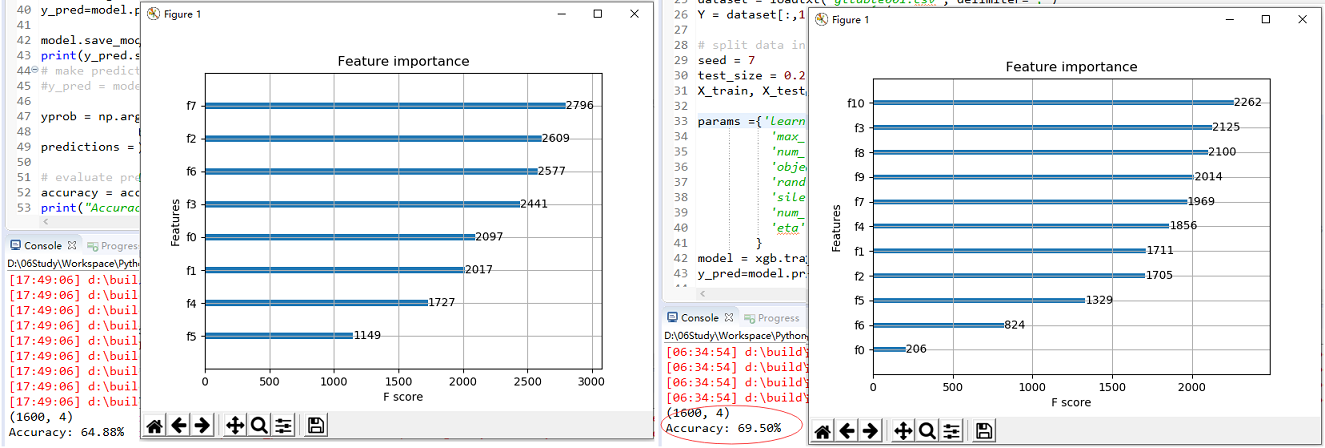看到“Feature Importance”图的代码如下所示：

# 显示重要特征
plot_importance(model)
plt.show()


## 4.2. 训练参数优化

数据预处理、特征工程、调参对Xgboost的效果有着非常重要的影响。
在这里只介绍Tree，重点是调优“learning_rate”学习率和“max_dept”构建树的深度，一般学习率中0.1 ~ 1间调整，而树的深度是随着数据量和维度增加而增加，我实验的经历是从5开始，已经到24了，再增大的效果是反而下降。网上推荐“max_dept”取值为6 ~ 10。

params ={'learning_rate': 0.4,
'max_depth': 20,                # 构建树的深度，越大越容易过拟合
'num_boost_round':2000,
'objective': 'multi:softprob', # 多分类的问题
'random_state': 7,
'silent':0,
'num_class':4,                 # 类别数，与 multisoftmax 并用
'eta':0.8                      #为了防止过拟合，更新过程中用到的收缩步长。eta通过缩减特征 的权重使提升计算过程更加保守。缺省值为0.3，取值范围为：[0,1]
}


关于优化，后续将参考寒小阳的博文《机器学习系列(12)_XGBoost参数调优完全指南（附Python代码）》使用xgb.cv()函数交叉验证，优化参数。

# 5. 模型训练的应用实践代码

'''
Created on 2019年4月1日

@author: xiaoyw
'''
from xgboost import plot_importance
from matplotlib import pyplot as plt

import xgboost as xgb
from sklearn.model_selection import train_test_split
from sklearn.metrics import accuracy_score
import numpy as np
# split data into X and y
X = dataset[:,:8]
Y = dataset[:,1]

# split data into train and test sets
seed = 7
test_size = 0.2
X_train, X_test, y_train, y_test = train_test_split(X, Y, test_size=test_size, random_state=seed)

params ={'learning_rate': 0.4,
'max_depth': 20,                # 构建树的深度，越大越容易过拟合
'num_boost_round':2000,
'objective': 'multi:softprob', # 多分类的问题
'random_state': 7,
'silent':0,
'num_class':4,                 # 类别数，与 multisoftmax 并用
'eta':0.8                      #为了防止过拟合，更新过程中用到的收缩步长。eta通过缩减特征 的权重使提升计算过程更加保守。缺省值为0.3，取值范围为：[0,1]
}
model = xgb.train(params,xgb.DMatrix(X_train, y_train))
y_pred=model.predict(xgb.DMatrix(X_test))

model.save_model('testXGboostClass.model')  # 保存训练模型

yprob = np.argmax(y_pred, axis=1)  # return the index of the biggest pro

predictions = [round(value) for value in yprob]

# evaluate predictions
accuracy = accuracy_score(y_test, predictions)
print("Accuracy: %.2f%%" % (accuracy * 100.0))

# 显示重要特征
plot_importance(model)
plt.show()


# 6. 关于fit（）与train（）的区别补充

这段是困惑的内容，以下是摘自网络参考文档，还未展开研究。

1、xgboost.XGBClassifier()利用函数参数设置模型参数
XGBClassifier()使用sklearn接口(推荐) ，XGBClassifier - 是xgboost的sklearn包。这个包允许我们像GBM一样使用Grid Search 和并行处理。

2、xgboost.train()利用param列表设置模型参数。
原始的xgboost，xgb - 直接引用xgboost。 有“cv”函数。

参考代码，还抽出时间实验，先收录到本文中再用。


clf = XGBClassifier(
n_estimators=20,  # 迭代次数
learning_rate=0.1,  # 步长
max_depth=5,  # 树的最大深度
min_child_weight=1,  # 决定最小叶子节点样本权重和
silent=1,  # 输出运行信息
subsample=0.8,  # 每个决策树所用的子样本占总样本的比例（作用于样本）
colsample_bytree=0.8,  # 建立树时对特征随机采样的比例（作用于特征）典型值：0.5-1
objective='multi:softmax',  # 多分类！！！！！！
num_class=3,
seed=27)
print "training..."
clf.fit(x_train, y_train, verbose=True)
fit_pred = clf.predict(x_test)
print fit_pred
xgb_params = {
'learning_rate': 0.1,  # 步长
'max_depth': 5,  # 树的最大深度
'objective': 'multi:softmax',
'num_class': 3,
'min_child_weight': 1,  # 决定最小叶子节点样本权重和，如果一个叶子节点的样本权重和小于min_child_weight则拆分过程结束。
'silent': 0,  # 输出运行信息
'subsample': 0.8,
'colsample_bytree': 0.8,  # 建立树时对特征随机采样的比例（作用于特征）典型值：0.5-1
'seed': 27}
print "training..."
model = xgb.train(xgb_params, xgb.DMatrix(x_train, y_train), num_boost_round=20)
train_pred = model.predict(xgb.DMatrix(x_test))
count = 0
print train_pred
# 判断train_pred和fit_pred是否一致
for i in np.arange(0, len(train_pred)):
if (train_pred[i] == fit_pred[i]):
count += 1
print "len:", len(train_pred)
print "count:", count
if count == len(train_pred):
print "true"
else:
print "false"


参考：
《XGBOOST从原理到实战：二分类 、多分类》 CSDN博客 DemonHunter211 2018年7月
《xgboost 多分类：objective参数（reg:linear,multi:softmax,multi:softprob）对比分析》 CSDN博客 phyllisyuell 2018年7月
《TensorFlow CNN卷积神经网络实现工况图分类识别（一）》 CSDN博客 肖永威 2019年3月
《xgboost.XGBClassifier， xgboost.train()》 CSDN博客 liulina603 2017年12月
《XGBoost算法应用入门学习实践》 CSDN博客 肖永威 2018年6月

展开全文机器学习 XGBoost Python 预测概率
• 本文以[CIFAR-10](https://www.cs.toronto.edu/~kriz/cifar.html)为数据集，基于Tensorflow介绍了CNN(卷积神经网络)图像分类模型的构建过程，着重分析了在建模过程中卷积层、池化层、扁平化层、全连接层、输出层的...Tensorflow 卷积 池化
• Spark随机森林之多分类模型关于随机森林随机森林算法是机器学习、计算机视觉等领域内应用极为广泛的一个算法，它不仅可以用来做分类，也可用来做回归即预测，随机森林机由多个决策树构成，相比于单个决策树算法，它...spark 机器学习
• 经典的机器学习建模数据是由特征列和单一目标列构成的，比如要做广告的CTR预测，其实模型关心的是一个广告曝光后是否会被点击，这是一个单一目标场景的建模过程。但是在实际应用场景中，往往有时候会出现“既要也要...机器学习
• 类别集(caegories)有(bird,dog,pig)三个类别，输入一张图片，分类模型会给图像分配个标签，每个标签的概率不相同，取最大作为其类别，这就完成了图像分类的任务 图像分类经典的分类模型 AlexNet AlexNet简介： ...计算机视觉
• 本文根据自己对分类模型的评价指标的理解以及其它博主的理解进行总结而成，有疑问或不对地方，请留言指出。 1. 什么是评价指标？ 评价指标：是针对 同份数据，不同算法模型 或者 同模型但不同模型参数，而给出这个...python 机器学习
• ## 文本分类——常见分类模型

万次阅读 多人点赞 2018-11-06 17:37:56
文本分类方法模型主要分为两个大类，一类是基于规则的分类模型；另一类是基于概率统计的模型。 基于规则的模型   基于规则的分类模型相对简单，易于实现。它在特定领域的分类往往能够取得较好的效果。相对于...自然语言处理 机器学习
• 怎么将二分类模型应用到多分类问题 二分类模型数量众多，但实际应用中往往待预测类别数量不只有2个，于是有了一些将二分类模型应用到多分类的方法。 常见二分类模型 One-vs-Rest One-vs-One Directed Acyclic ...
• 对于二分类模型，我们通常可以使用ROC曲线来评估模型的预测效果。这里，我们介绍一下在多分类中衡量模型评估准确度的一种方法–kappa系数评估方法。 Kappa系数 首先，我们介绍一下kappa系数： kappa系数是用在...
• ## 机器学习中最常见的四种分类模型

万次阅读 多人点赞 2020-05-23 12:30:00
点击蓝字关注我，有干货领取！作者：Jason Brownlee翻译：候博学前言 机器学习是一个从训练集中学习出算法的研究领域。分类是一项需要使用机器学习算法的任务，该算法学习如何为数据集...
• 机器学习中的分类模型有逻辑回归、朴素贝叶斯、决策树、支持向量机、随机森林、梯度提升树等分类算法，不仅可以进行二分类，还可以进行多分类。 一、逻辑回归 逻辑回归的本质就由线性回归演变而来，是一个线性分类器...机器学习
• NLP——文本分类模型（一） 1、引入 最为自然语言处理中最为基础的任务，文本分类一直受到了很的关注，本文主要关注在深度学习在文本分类中的应用。通过textCNN，DCNN，RCNN，HAN四种经典的文本分类模型来描述深度...
• 需求描述：基于构建简单的多分类模型需求，使用的是keras深度学习库实现的，实现代码简单可用，模型优点是训练速度快，准确率达97%，适合粗粒度分类。 实现步骤：1、预处理数据：包括构建自定义词典、分词、去停用...深度学习 tensorflow
• 一直想写篇度量指标的文章，梳理一下这块的知识点，可能想了太多次，总以为自己已经写过了，今天看文章列表，竟然没有相关的内容，刚好最近在做多分类模型，借此机会整理一下。 混淆矩阵（confusion matrix） 在...机器学习
• 如果理解ROC曲线的话，就很容易理解KS了。roc横纵坐标分别为FPR、TPR。...征信模型中，最期望得到的信用分数分布是正态分布，对于正负样本分别而言，也都是期望呈正态分布的样子。如果KS值过大，一般...
• ## keras的分类模型

千次阅读 2018-06-05 13:17:11
基于keras的神经网络分类模型（二分类、多分类）from matplotlib import pyplot import pandas as pd from sklearn.preprocessing import MinMaxScaler from keras.models import Sequential from keras.layers.core...
• 随着层数越来越，梯度在多层传播时会越来越小，直到梯度消失，于是随着层数越来越，训练误差会越来越大。 ResNet模型的动机是要解决网络层次比较深时无法训练的问题，借鉴了高速网络模型的思想，设计出了一个...机器学习 深度学习 神经网络 人工智能 图像处理
• 注意：准确率这一指标在Unbalanced数据集上的表现很差，因为如果我们的正负样本数目差别很大，比如正样本100个，负样本9900个，那么直接把所有的样本都预测为负， 准确率为99%，但是此分类模型实际性能是非常差的，...评价指标
• 【火炉炼AI】机器学习011-分类模型的评估：准确率，精确率，召回率，F1值 【本文所使用的Python库和版本号】: Python 3.5, Numpy 1.14, scikit-learn 0.19, matplotlib 2.2   在前面的（【火炉炼AI】机器学习004...机器学习 人工智能 数据挖掘
• R语言有序多分类Logistic回归模型实战 目录 R语言有序多分类Logistic回归模型实战： #导入包 #数据加载 #有序多分类Logistic回归模型 #导入包 #程序包MASS提供polr()函数可以进行ordered logit或probit...
• 模型进行评估时，可以选择很种指标，但不同的指标可能得到不同的结果，如何选择合适的指标，需要取决于任务需求。 针对二元分类结果，常用的评估指标有如下三个：查准率（Precision）、查全率（Recall）以及F-...
• 多分类模型和多任务模型（Multi-task Model）的区别在于： 多分类模型：样本集包含多个类别，但是一个样本只属于一类。 多任务模型：样本集包含多个类别，一个样本可以属于多个类别。 一、多分类模型 1、多分类...multi-task
• 上一篇文章中，提到正确率(Accuracy)是机器学习分类任务中过得一个模型评估方法，并指出正确率(Accuracy)虽然简单直观，但在很时候并不是一个真正正确的评估指标。那还有什么评估方法呢?我们还是举地震、癌症、...机器学习
• ## 分类模型

千次阅读 2020-11-05 14:53:16
分类模型：概率生成模型 概念 分类是寻找一个函数，当输入一个对象，输出为该对象所属的类别。 输入数值化 对于分类问题来说，要把一个对象当作一个函数的输入，则需要对对象进行数值化操作。 特征数值化：用以组...机器学习
• softmax回归模型为logistic回归模型多分类问题上的推广。 假设我们有一个数组V，Vi是V中的第i个元素，那么这个元素的softmax值就是： 也就是说，是该元素的指数与所有元素指数和的比值。 由该公式我们可计算...softmax 回归...